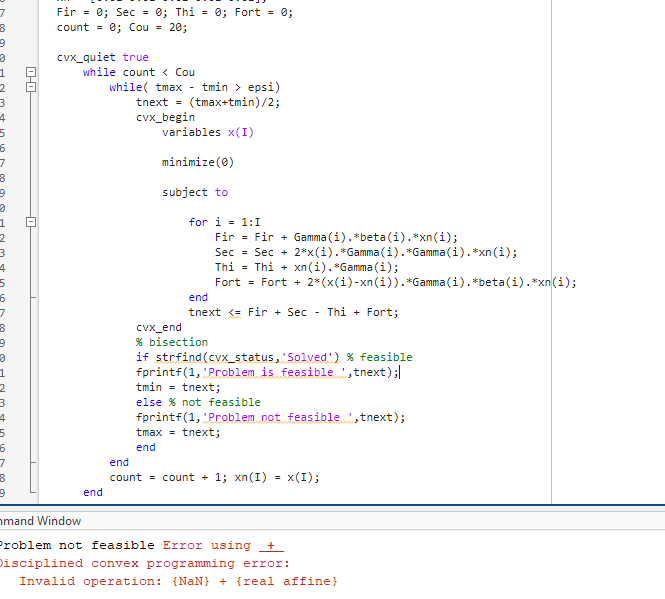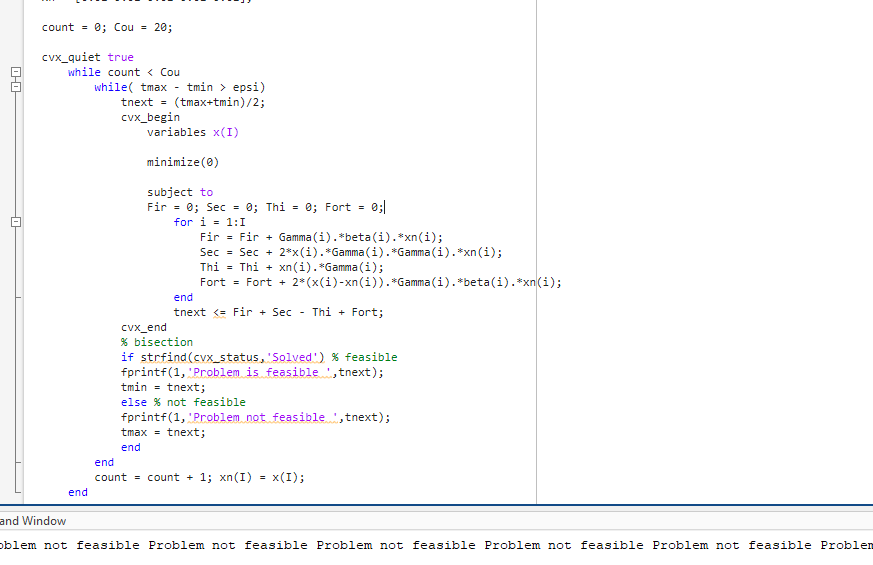# The reason of causing 'NaN' value in CVX feasibility solve

## Hello guys, I am new here and it’s the first time to use the CVX tool. I met a problem when I was doing my model. I have fixed it but do not understand why it happens.Question_1665×599 21.2 KBQuestion_2873×565 18.8 KB In figure 1, the command window shows a ‘NaN’ value so the program can not process. When I move the base settings from the outside-CVX part into the inside-CVX part, the ‘NaN’ value disappear and the codes can work. I have no idea why this can help because I think the settings for the base values might not influence much if it is in CVX or outside. Thank you for telling me the reason or tell me which part in the CVX handbook might answer the question.

And for my formula above is a Taylor first-order expansion. The xn(I) is a pre-set vector. Gamma(I) and beta(I) are already known. The reason why I use Fir, Sec, Thi and Fort is I want to sum the values as the written euqation below. I think the program does not wrong, it can find the final x(I) and the max-min result.

When the problem is infeasible, the variable value `x` is a vector of `NaN`, which causes `xn` to be set to `NaN`, which is used as input data in the next CVX problem. CVX considers `NaN` in input data to be invalid`, hence the error message. Algorithm 4.1 of Convex Optimization – Boyd and Vandenberghe shows how to correctly implement the bisection algorithm.

1 Like

Thank you Mark. So if the problem is infeasible, how does the program process in the next count with the “NaN” value of xn(I)? The codes can work when I move the default settings of Fir, Sec, Thi and Fort = 0 into the CX part, so the code works by using them in “0” value? Thank you.

When CVX encounters `NaN` in the input data, CVX considers it to be `{invalid}`, and issues an error message and does not call the solver. That’s what I tried to explain in my previous post.

I know. But when I set a default value 0 (as shown in figure 2), the program and work. I wonder why it can work. Does the codes would treat the value in 0 and do the next iteration?

When you start with a different problem, the results of that problem will be different. Apparently with this other starting value, each problem is solved to optimality, and hence never produces `NaN.

Why don’t you fix your bisection algorithm implementation, as I previously suggested?

I have checked the Algorithm 4.1 you sent to me and then repair it. Sorry I make the opposite settings. When it’s feasible, the max should be set to the middle value, not the min.
That’s weird tbh since I study the from github…maybe that’s how they avoid Plagiarism.

But I am still wonder how the codes work when in the constraint there are “NaN” values inside. Since the Taylor expasion method I use requires previous iteration result value and they are NaN as I set xn(I) = x(I).

Not everything on github is correct or good. Anyone can post anything.

“Convex Optimization” by Boyd and Vandenberghe is a very highly regarded book which has been closely scrutinized by many people.

You shouldn’t start with any `NaN`. Nor should you ever set anything to `NaN`. At each iteration, either the lower bound or upper bound is updated to the midpoint of the existing lower and upper bound. if neither bound starts at `NaN`, you will never update anything to `NaN`.

1 Like

However,perhaps I don’t really know what you’re doing, and you’re trying to combine SCA with bisection, which I doubt makes any sense.

As for SCA, see

1 Like

Thank you for the referenced link. My aim of the program is to find the max-min value of the answer. The original problem is non-convex so I use the Taylor expansion to convert it into a quasi-convex which required bisection to be solved.
What makes me confused now is that the Taylor expansion reuires the result of the previous iteration. So I wonder if the result of the current iteration is infeasible, which is ‘NaN’ to the codes, how will the program consider the next xn(I) value for the next iteration.
The method I use in the codes is from Algorithm 1 in this paper.

This apparently is a hierarchical algorithm, where the outer level is iteration SCA, and the inner iteration is using bisection to solve the optimization problem in that iteration of SCA. Each instance of the bisection algorithm (one instance per SCA iteration) requires solving several (inner iteration) feasibility problems

I think you are supposed to solve each instance of the bisection algorithm to
optimality, and use the optimal solution as input to the next SCA iteration, which will have a new instance of the bisesction algorithm The infeasibility of various (inner problem) feasibility problems solved as part of the bisection algorithm is just to determine which of lower and upper bound gets changed for the next bisection (inner) feasibility problem, all within that SCA iteration. Only when the bisection algorithm has concluded, is the input updated to create the (outer) problem for the next SCA iteration…

As to whether each (outer) problem in SCA solved by bisection is actually feasible and makes the overall algorithm work, I have no idea.

1 Like

So that the inner iteration by using bisection should generate a feasible value (with tmax -tmin < epsi), reset the tmax and tmin, and then set the feasibe solution of x(I) = xn(I) for the next outer iteration by SCA, That makes sense. Thank you.

Maybe that should be called SQCA.

1 Like